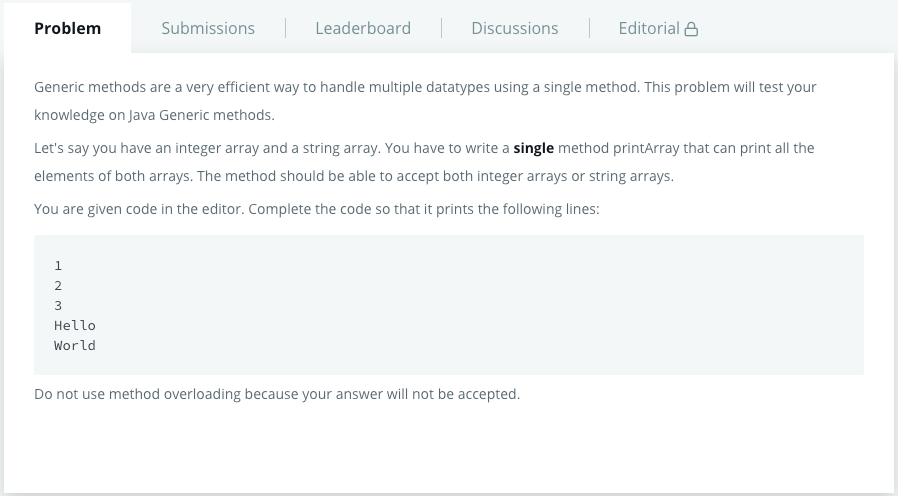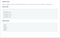# 07/15/2020Photo by Lex Sirikiat on Unsplash

## Java GenericsJava Generics, retrieved from https://www.hackerrank.com/challenges/java-generics/problem
`import java.io.IOException;import java.lang.reflect.Method;class Printer{    //Write your code here    public void printArray(T[] elements){        for (T element: elements){            System.out.println(element);        }    }}public class Solution {    public static void main( String args[] ) {        Printer myPrinter = new Printer();        Integer[] intArray = { 1, 2, 3 };        String[] stringArray = {"Hello", "World"};        myPrinter.printArray(intArray);        myPrinter.printArray(stringArray);        int count = 0;        for (Method method : Printer.class.getDeclaredMethods()) {            String name = method.getName();            if(name.equals("printArray"))                count++;        }        if(count > 1)System.out.println("Method overloading is not allowed!");    }}`
`import java.io.IOException;import java.lang.reflect.Method;class Printer{    //Write your code here    <T>void printArray(T[] elements){        for (T element: elements){            System.out.println(element);        }    }}public class Solution {    public static void main( String args[] ) {        Printer myPrinter = new Printer();        Integer[] intArray = { 1, 2, 3 };        String[] stringArray = {"Hello", "World"};        myPrinter.printArray(intArray);        myPrinter.printArray(stringArray);        int count = 0;        for (Method method : Printer.class.getDeclaredMethods()) {            String name = method.getName();            if(name.equals("printArray"))                count++;        }        if(count > 1)System.out.println("Method overloading is not allowed!");    }}`

# 07/28/2020

## Java SortJava Sort, Retrieved from https://www.hackerrank.com/challenges/java-sort/problem
`import java.util.*;class Student{    private int id;    private String fname;    private double cgpa;    public Student(int id, String fname, double cgpa) {        super();        this.id = id;        this.fname = fname;        this.cgpa = cgpa;    }    public int getId() {        return id;    }    public String getFname() {        return fname;    }    public double getCgpa() {        return cgpa;    }}//Complete the codepublic class Solution{    public static void main(String[] args){        Scanner in = new Scanner(System.in);        int testCases = Integer.parseInt(in.nextLine());        List<Student> studentList = new ArrayList<Student>();        while(testCases>0){            int id = in.nextInt();            String fname = in.next();            double cgpa = in.nextDouble();            Student st = new Student(id, fname, cgpa);            studentList.add(st);            testCases--;        }        Collections.sort(studentList, Comparator.comparing(Student::getCgpa).reversed().thenComparing(Student::getFname).thenComparing(Student::getId));        for(Student st: studentList){            System.out.println(st.getFname());        }    }}`

## Extra words:

Undergraduate student / Research assistant/ Always curious / Opinions are mine

## More from Andrew Chen

Undergraduate student / Research assistant/ Always curious / Opinions are mine